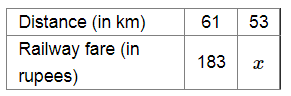# The railway fare for 61 km is Rs 183.

Question:

The railway fare for 61 km is Rs 183. Find the fare for 53 km.

Solution:

Let Rs x be the railway fare for a journey of distance 53 km.The lesser the distance, the lesser will be the fare.

So, it is a case of direct proportion .

Now, $\frac{61}{183}=\frac{53}{x}$

$\Rightarrow x=\frac{53 \times 183}{61}$

$\Rightarrow x=159$

The railway fare for a journey of distance 53 km is Rs 159.